Definitions of Square Dance Calls and Concepts

Index -->  Plus  |  A1  |  A2  |  C1  |  C2  |  C3A  |  C3B  |  C4  |  NOL  |
Definitions (Text Only) -->  Plus  |  A1  |  A2  |  C1  |  C2  |  C3A  |  C3B  |  C4  |  NOL  |
 Find call:

 \$B8@8l(B view (admin)

\$BE,@Z\$J0lHL2=\$5\$l\$?(B 1/4 Tag \$B\$+\$i!%(B

Very Centers \$B\$,Counter Rotate 1/4 (\$B30B&\$r2s\$k(B) \$B\$r\$7\$F(B, each side \$B\$N(B Centers \$B\$,(B Hinge \$B\$r\$7(B, Centers \$B\$,(B Star Circulate \$B\$r\$9\$k4V\$K(B Outsides \$B\$O(B Trade \$B\$r\$7(B, each side \$B\$N(B Centers \$B\$,(B Cast Off 3/4 \$B\$r\$9\$k4V\$KB>\$N?M\$O(B Phantom Hourglass Circulate \$B\$r\$7\$^\$9!%(B

\$BJ?9T\$J(B Line \$B\$G=*\$o\$j\$^\$9!%(B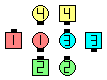Cross Chain Reaction\$B\$NA0(B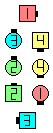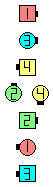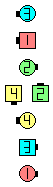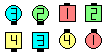Very Centers and Diagonally-Facing dancers Diagonal Pass Thru as End of Center Line Counter Rotate 1/4\$B\$N8e(B Each side Centers Hinge\$B\$N8e(B Centers Star Circulate as Outsides Trade\$B\$N8e(B Center Line Cast Off 3/4 as Others move up\$B\$N8e(B (\$B=*\$o\$j(B)

Cross Chain Reaction \$B\$O(B Chain Reaction [A1,C1] \$B\$HF1\$8\$G\$9\$,(B, Very Centers \$B\$,\$0\$G\$O\$J\$/(B) \$B30B&\$N?M\$H(B Diagonal Pass Thru \$B\$9\$kE@\$,0[\$J\$j\$^\$9!%(B

\$B%R%s%H(B: Diagonal Pass Thru \$B\$r\$9\$k?M\$O(B, \$B\$*8_\$\$\$KF0\$/A0\$K(B point \$B\$9\$Y\$-\$G\$9!%(B \$B\$5\$i\$K(B, \$B30B&\$N?M\$G(B Diagonal Pass Thru \$B\$r\$7\$J\$\$?M\$O(B, center \$B\$+\$i(B Diagonal Pass Thru \$B\$7\$F\$/\$k?M\$,NY\$KMh\$k\$^\$GF0\$\$\$F\$O\$\$\$1\$^\$;\$s!%(B \$B\$^\$?(B, Hinge \$B\$rM=A[\$7\$FAa\$^\$C\$FF0\$\$\$F\$7\$^\$&\$H(B, \$B<+J,\$b\$=\$7\$FB>\$N?M\$b(B, \$B0LCV\$r4V0c\$(\$F\$7\$^\$&\$3\$H\$K\$J\$k\$G\$7\$g\$&!%(B

anything Cross Reaction [C3A]: anything Reaction [C3A] \$B\$r8+\$F\$/\$@\$5\$\$!%(B

Cross Chain Reaction But anything [C3AV]: Centers \$B\$O:G8e\$N(B Cast Off 3/4 \$B\$r(B anything call \$B\$G(B replace \$B\$7\$^\$9!%(BCALLERLAB definition for Cross Chain ReactionChoreography for Cross Chain ReactionComments? Questions? Suggestions?

https://www.ceder.net/def/crosschainreaction.php?language=japan&level=C3A
10-April-2021 08:31:25
Copyright © 2021 Vic Ceder.  All Rights Reserved.